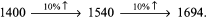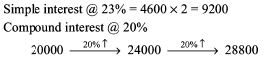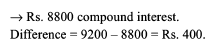Courses

# Test: SI & CI- 3

## 15 Questions MCQ Test Quantitative Aptitude (Quant) | Test: SI & CI- 3

Description
This mock test of Test: SI & CI- 3 for CAT helps you for every CAT entrance exam. This contains 15 Multiple Choice Questions for CAT Test: SI & CI- 3 (mcq) to study with solutions a complete question bank. The solved questions answers in this Test: SI & CI- 3 quiz give you a good mix of easy questions and tough questions. CAT students definitely take this Test: SI & CI- 3 exercise for a better result in the exam. You can find other Test: SI & CI- 3 extra questions, long questions & short questions for CAT on EduRev as well by searching above.
QUESTION: 1

### A sum of Rs. 8000 is borrowed at 5% p.a. compound interest and paid back in 3 equal annual instalments. What is the amount of each instalment?

Solution:

Let the repayment annually be X. Then: 8000 + 3 years interest on 8000 (on compound interest of 5%) = X + 2 years interest on X + X + 1 years interest on X + X → X = 2937.67

QUESTION: 2

### A person lent out some money for 1 year at 6% per annum simple interest and after 18 months, he again lent out the same money at a simple interest of 24% per annum. In both the cases, he got Rs. 4704. Which of these could be the amount that was lent out in each case if interest is paid half-yearly?

Solution:

4200 + (4 % of 4200) 3 times = 4200 + 0.04 x 3 x 4200 = 4704.

QUESTION: 3

### Hans Kumar borrows Rs. 7000 at simple interest from the village moneylender. At the end of 3 years, he again borrows Rs. 3000 and closes his account after paying Rs. 4615 as interest after 8 years from the time he made the first borrowing. Find the rate of interest.

Solution:

The interest would be paid on 7000 for 3 years + 10000 for 5 years. @ 6.5% the total interest for 8 years = 1365 + 3250
= Rs. 4615

QUESTION: 4

An amount of Rs. 12820 due 3 years hence, is fully repaid in three annual instalments starting after 1 year. The first instalment is 1/2 the second instalment and the second instalment is 2/3 of the third instalment. If the rate of interest is 10% per annum, find the first instalment.

Solution:

Solve using options. Option (c) fits the situation as: 12820 = 2000 + 2 years interest on 2000 + 4000 +1 years interest on 4000 + 6000 (use 10% compound interest for calculation of interest) → 12820 = 2000 + 420 + 4000 + 400 + 6000.
Thus, option (c) fits the situation perfectly.

QUESTION: 5

Michael Bolton has \$90,000 with him. He purchases a car, a laptop and a flat for \$15,000, \$13,000 and \$35,000 respectively and puts the remaining money in a bank deposit that pays compound interest @15% per annum. After 2 years, he sells off the three items at 80% of their original price and also withdraws his entire money from the bank by closing the account. What is the total change in his asset?

Solution:

The final value would be: 0.8 x 63000 + 27000 x 1.15 x 1.15 = 86107.5. →Drop in value = 4.32%

QUESTION: 6

Read the following and answer the questions that follow. 4. A certain sum of money was lent under the following repayment scheme based on Simple Interest: 8% per annum for the initial 2 years 9.5% per annum for the next 4 years 11% per annum for the next 2 years 12% per annum after the first 8 years Find the amount which a sum of Rs. 9000 taken for 12 years becomes at the end of 12 years.

Solution:

9000 + 720 + 720 + 855 + 855 + 855 + 855 + 990
+ 990 + 1080 + 1080 + 1080 + 1080
= 9000 + 720 x 2 + 855 x 4 + 990 x 2 + 1080 x 4 = 20160

QUESTION: 7

Mr. X, a very industrious person, wants to establish his own unit. For this he needs an instant loan of Rs. 5,00,000 and, every five years he requires an additional loan of Rs. 100,000. If he had to clear all his outstandings in 20 years, and he repays the principal of the first loan equally over the 20 years, find what amount he would have to pay as interest on his initial borrowing if the rate of interest is 10% p.a. Simple Interest.

Solution:

The simple interest would be defined on the basis of the sum of the AR

50000 + 47500 + 45000 + ... + 2500 = 525000.

QUESTION: 8

Interest obtained on a sum of Rs. 5000 for 3 years is Rs. 1500. Find the rate percent.

Solution:

The interest earned per year would be 1500/3=500. This represents a 10% rate of interest.

QUESTION: 9

Rs. 1694 is repaid after two years at compound interest. Which of the following is the value of the principal and the rate?

Solution:QUESTION: 10

What is the difference between compound interest and simple interest for the sum of Rs. 20,000 over a 2 year period if the compound interest is calculated at 20% and simple interest is calculated at 23%?

Solution:QUESTION: 11

A sum was invested at simple interest at a certain interest for 2 years. It would have fetched Rs. 60 more had it been invested at 2% higher rate. What was the sum?

Solution:

Based on the information we have, we can say that there would have been Rs. 30 extra interest per year. For 2% of the principal to be equal to Rs. 30, the principal amount should be Rs. 1500

QUESTION: 12

In what time will the simple interest on Rs. 1750 at 9% per annum be the same as that on Rs. 2500 at 10.5% per annum in 4 years?

Solution:

42% on 2500 = Rs. 1050. The required answer would be: 1050/157.5 = 6 years and 8 months.

QUESTION: 13

What annual payment will discharge a debt of Rs. 808 due in 2 years at 2% per annum?

Solution:

A x (1.02) +A = 808 x (1.02)2 → A = Rs. 400

QUESTION: 14

If the compound interest on a certain sum for 2 years is Rs. 21. What could be the simple interest?

Solution:

If we take the principal as 100, the Cl @ 10% Rate of interest would be Rs. 21. In such a case, the SI would be Rs. 20.

QUESTION: 15

If the difference between compound and simple interest on a certain sum of money for 3 years at 2% p.a. is Rs. 604, what is the sum?

Solution:

Solve through trial and error using the values of the options. Option (a) 500000 fits the situation perfectly as the SI = Rs. 30000 while the Cl = 30604.